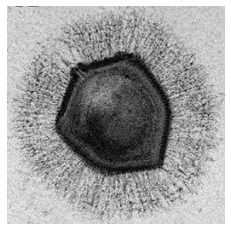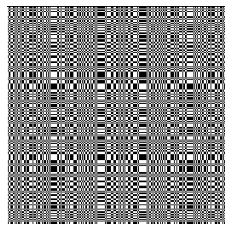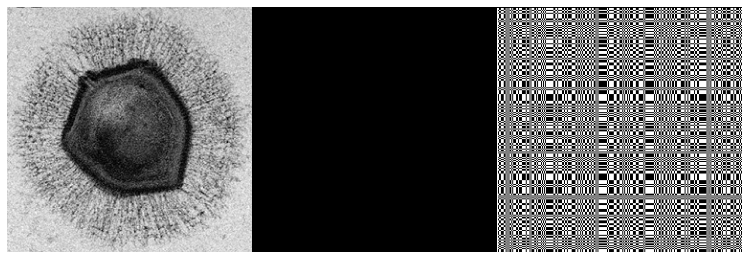# HoloML in Stan: Low-photon Image Reconstruction¶

### Brian Ward, Bob Carpenter, and David Barmherzig¶

#### June 13, 2022¶

This case study is a reimplementation of the algorithm described in Barmherzig and Sun (2022)  as a Stan model. This requires the new features available in Stan 2.30

## Introduction¶

The HoloML technique is an approach to solving a specific kind of inverse problem inherent to imaging nanoscale specimens using X-ray diffraction.

To solve this problem in Stan, we first write down the forward scientific model given by Barmherzig and Sun, including the Poisson photon distribution and censored data inherent to the physical problem, and then find a solution via penalized maximum likelihood.

### Experimental setup¶

In coherent diffraction imaging (CDI), a radiation source, typically an X-ray, is directed at a biomolecule or other specimen of interest, which causes diffraction. The resulting photon flux is measured by a far-field detector. The expected photon flux is approximately the squared magnitude of the Fourier transform of the electric field causing the diffraction. Inverting this to recover an image of the specimen is a problem usually known as phase retrieval. The phase retrieval problem is highly challenging and often lacks a unique solution .

Holographic coherent diffraction imaging (HCDI) is a variant in which the specimen is placed some distance away from a known reference object, and the data observed is the pattern of diffraction around both the specimen and the reference. The addition of a reference object provides additional constraints on this problem, and transforms it into a linear deconvolution problem which has a unique, closed-form solution in the idealized setting .

The idealized version of HCDI is formulated as

• Given a reference $R$, data $Y = | \mathcal{F}( X + R ) | ^2$
• Recover the source image $X$

Where $\mathcal{F}$ is an oversampled Fourier transform operator.

However, the real-world set up of these experiments introduces two additional difficulties. Data is measured from a limited number of photons, where the number of photons received by each detector is modeled as Poisson distributed with expectation given by $Y_{ij}$ (referred to in the paper as Poisson-shot noise). The expected number of photons each detector receives is denoted $N_p$. We typically have $N_p < 10$ due to the damage that radiation causes the biomolecule under observation. Secondly, to prevent damage to the detectors, the lowest frequencies are removed by a beamstop, which censors low-frequency observations.

The maximum likelihood estimation of the model presented here is able to recover reasonable images even under a regime featuring low photon counts and a beamstop.

## Simulating Data¶

We simulate data from the generative model directly. This corresponds to the approach taken by Barmherzig and Sun, and is based on MATLAB code provided by Barmherzig.

#### Imports and helper code¶

Generating the data requires a few standard Python numerical libraries such as scipy and numpy. Matplotlib is also used to simplify loading in the source image and displaying results.

In :
import numpy as np
from scipy import stats
import cmdstanpy

import matplotlib as mpl
import matplotlib.image as mpimg
import matplotlib.pyplot as plt

def rgb2gray(rgb):
"""Convert a nxmx3 RGB array to a grayscale nxm array.

This function uses the same internal coefficients as MATLAB:
https://www.mathworks.com/help/matlab/ref/rgb2gray.html
"""
r, g, b = rgb[:, :, 0], rgb[:, :, 1], rgb[:, :, 2]
gray = 0.2989 * r + 0.5870 * g + 0.1140 * b

return gray

Extra display settings set, omitted from rendered case study

Out:

#### Simulation parameters¶

To match the figures in the paper (in particular, Figure 9), we use an image of size 256x256, $N_p = 1$ (meaning each detector is expected to receive one photon), and a beamstop of size 25x25 (corresponding to a radius of 13), and a separation d equal to the size of the image.

In :
N = 256
d = N
N_p = 1
r = 13

M1 = 2 * N
M2 = 2 * (2 * N + d)


We can then load the source image used for these simulations. In this model, the pixels of $X$ grayscale values represented on the interval [0, 1]. A conversion is done here from the standard RGBA encoding using the above rgb2gray function.

The following is a picture of a giant virus known as a mimivirus.

Image credit: Ghigo E, Kartenbeck J, Lien P, Pelkmans L, Capo C, Mege JL, Raoult D., CC BY 2.5, via Wikimedia Commons

In :
X_src = rgb2gray(mpimg.imread('mimivirus.png'))
plt.imshow(X_src, cmap='gray', vmin=0, vmax=1)

Out:
<matplotlib.image.AxesImage at 0x7efe104af4c0>The pattern used here is known as a uniformly redundant array (URA) . It has been shown to be an optimal reference image for this kind of work, but other references (including none at all) could be used with the same Stan model.

The code used to generate this grid is omitted from this case study. Various options such as cappy exist to generate these patterns in Python.

In :
R = np.loadtxt('URA.csv', delimiter=",", dtype=int)
plt.imshow(R, cmap='gray')

Out:
<matplotlib.image.AxesImage at 0x7efe083943a0>We create the specimen-reference hybrid image by concatenating the $X$ image, a matrix of zeros, and the reference $R$. In the true experiment, this is done by placing the specimen some distance d away from the reference, with opaque material between.

This distance is typically the same as the size of the specimen, N. One contribution of the HoloML model is allowing recovery with the reference placed closer to the specimen, and the Stan model allows for this as well.

For this simulation we use the separation of d = N.

In :
X0R = np.concatenate([X_src, np.zeros((N,d)), R], axis=1)
plt.imshow(X0R, cmap='gray')

Out:
<matplotlib.image.AxesImage at 0x7efe083da860>We can simulate the diffraction pattern of photons from the X-ray by taking the absolute value squared of the 2-dimensional oversampled FFT of this hybrid object.

The oversampled FFT (denoted $\mathcal{F}$ in the paper) corresponds to padding the image in both dimensions with zeros until it is a desired size. For our case, we define the size of the padded image, M1 by M2, to be two times the size of our hybrid image, so the resulting FFT is twice oversampled. This is the oversampling ratio traditionally used for this problem, however Barmherzig and Sun also showed that this model can operate with less oversampling as well.

In :
Y = np.abs(np.fft.fft2(X0R, s=(M1, M2))) ** 2
plt.imshow(np.fft.fftshift(np.log1p(Y)), cmap="viridis")

Out:
<matplotlib.image.AxesImage at 0x7efe06a3d7e0>